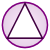Games
Problems
Go Pro!

# Prisms and Cylinders

Pro Problems > Math > Geometry > Solids > Prisms and Cylinders

# Featured Pro ProblemsFigure out the volume of a wedge of cheese cut from a wheelCalculate the dimensions of the room, given a cubic equation about its volumeCalculate the dimensions of a box, given some information about its volume and dimensionsCalculate the sum of the dimensions of a rectangular prism given its interior diagonal length and surface areaA rectangular prism volume problem involving a cubic equationCalculate the dimensions of the given box, given information about its volume relative to a second box

# Full Directory Listing

Box Dimensions, Cubic Box, Cutting the Cheese, Interior Diagonal, Room Volume, Two BoxesLike us on Facebook to get updates about new resources